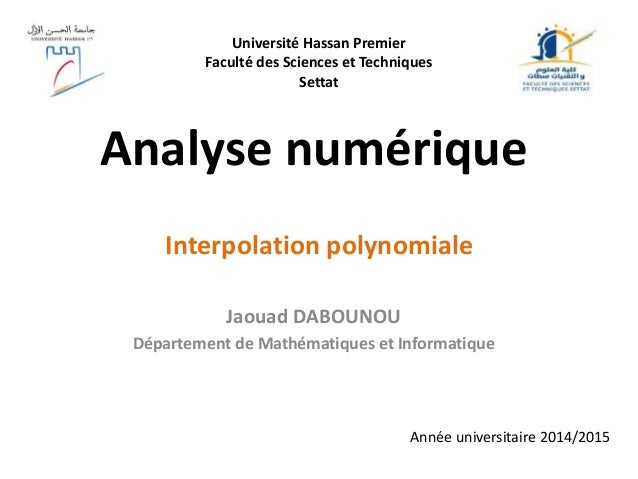Successfully reported this slideshow.Upcoming SlideShare
×

# Analyse numérique interpolation

3,348 views

Published on

Ce cours introduit l'interpolation polynomiale de Lagrange. Il fait partie du module d'analyse numérique donné en Parcours MIP à la FST de Settat, Université Hassan 1er.

Published in: Education
• Full Name
Comment goes here.

Are you sure you want to Yes NoAre you sure you want to  Yes  No

Are you sure you want to  Yes  No

### Analyse numérique interpolation

1. 1. Analyse numérique Jaouad DABOUNOU Département de Mathématiques et Informatique Interpolation polynomiale Année universitaire 2014/2015 Université Hassan Premier Faculté des Sciences et Techniques Settat
2. 2. Interpolation polynomiale • Introduction • Interpolation polynomiale • Le polynôme de Lagrange • Le polynôme de Newton • Algorithme de Neville • Interpolation par une fonction spline • Fonctions splines quadratiques • Fonctions splines cubiques
3. 3. Introduction Supposons que nous connaissons les valeurs d'une fonction en un nombre de points x0, x1, ... , xn, mais que nous n'avons pas l'expression analytique de f. Pour estimer la valeur de f en un point quelconque x  R, on peut construire un polynôme P tel que P(xi) = f(xi) pour i=0,1,...,n et utiliser l'approximation P(x)  f(x). C'est ce qu'on appelle interpolation polynomiale.
4. 4. Polynôme d’interpolation de Lagrange Théorème: Etant donnés n+1 points x0, x1, ... , xn, distincts et n+1 réels y0, y1, ... , yn, , il existe un polynôme PPn(R) et un seul tel que P(xi) = yi ; i=0,1, ... ,n )()( 0 xLyxP n i ii 
5. 5. Reque: Les Li vérifient les propriétés suivantes: Li(xi) = 1 Li(xj) = 0 si i  j Démonstration : Existence
6. 6. Démonstration :Démonstration : Unicité si on a deux polynômes P et Q ayant pour les n+1 points (xi , yi) P(xi) = Q(xi) = yi alors soit R = P – Q, on a : R(xi) = 0 pour les n+1 nombres distincts xi, i=0,n; or R, comme P et Q est de degré au maximum égal à n. On en déduit que R = 0.
7. 7. Interpolation polynomiale Exemple:On considère le tableau des xi etyi: i 0 1 2 3 4 xi -2 -1 0 1 2 yi 7,216 7,07 5,246 3,05 2,629 -2 -1.5 -1 -0.5 0 0.5 1 1.5 2 2.5 3 3.5 4 4.5 5 5.5 6 6.5 7 7.5 Points d’interpolation
8. 8. Interpolation polynomiale Exemple:Le polynôme d'interpolation de Lagrange en ces xi et yi est P(x) = 0.0348 x4 + 0.2879 x3 - 0.22 x2 - 2.298 x + 5.246 -2 -1.5 -1 -0.5 0 0.5 1 1.5 2 2 3 4 5 6 7 8
9. 9. Interpolation polynomiale Exemple: On considère la fonction On peut remarquer que P(xi)= f(xi) pour i=0,4
10. 10. Interpolation polynomiale Définition : Soit f une fonction définie aux points xi, supposés deux à deux distincts. On définit les différences divisées par récurrence comme suit :
11. 11. Interpolation polynomiale Définition : On considère sur l’intervalle [1 , 2] la fonction On considère les points xi = 1, et 2. Les coefficients du polynôme de Newton sont donnés par : x xf 1 )(  3 5 , 3 4
12. 12. Interpolation polynomiale Proposition : Démonstration : Par récurrence.
13. 13. Interpolation polynomiale Théorème: (Formule de Newton) Le polynôme d'interpolation de f aux points x0, x1, …, xn s'exprime dans la base de Newton comme: Démonstration : Par récurrence.
14. 14. Calcul de l'erreur Soit f:[a , b]  R une fonction donnée, on construit le polynôme P(x) qui interpole les valeurs de f aux points x0, x1, ... , xn (xi  [a , b]), ce qui conduit à yi = f(xi) pour i = 1, 2, ..., n. Soit e(x) l'erreur d'interpolation : e(x) = f(x) - P(x) Posons Int(x, x0 , x1, ... xn) le plus petit intervalle fermé contenant x, x1 , x2, ... xn et  la fonction (x) = (x – x0) (x – x1)..... (x - xn) Théorème 1.2. Quelque soit x[a , b], il existe   Int(x, x0 , x1, ... xn) tel que
15. 15. Algorithme de Neville Soit P0 l'unique polynôme de degré 0 qui passe par le point (x0,y0). De la même manière, on construit les polynômes P1, P2, ... Pn. Soit P01 l'unique polynôme de degré 1 passant par les points (x0,y0) et (x1 , y1). On définit aussi les polynômes P12, P23, ... P(n-1)n. De proche en proche on arrive au polynôme de plus grand degré P01...n qui coïncide avec l'unique polynôme d'interpolation P.
16. 16. Algorithme de Neville L'algorithme de Neville permet de construire par récurrence les polynômes Pi(i+1)...(i+m). Soit